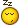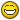Breaking polylines in the vertices

Recommended PostsIt would be great if someone can help me!

I need to break several polylines with different widths in each segment. If I explode them, I'll loose the widths, having just lines as a result. I'd like to separate each segment in the vertices. Is it possible with a lisp routine?

I couldn't find anything like that. I have a very little knowledge about lisp routines. I can build simple ones and I believe this one is not so simple, if it's possible, of course :wink:..

Thanks a lot!

Share on other sitesCouldn't sleep... not a whole lot of testing.

See if this works for you

;;; Break pline @ vertices LPS 2010-04-01
(defun c:test (/ idx obj endparam ptlst)
(setq temperr *error*)
(setq *error* errortrap)
(setq obj (vlax-ename->vla-object (car (setq ent (entsel "\nSelect polyline: ")))) )
(if ; test if polyline
(/= (vlax-get-property obj 'ObjectName) "AcDbPolyline")
(princ "\nSelected entity is not a polyline")
)
(setq ptlst (list (vlax-curve-getStartPoint obj))
idx 1)
(if (zerop (vlax-get obj 'Closed))
(setq endparam (vlax-curve-getParamAtPoint obj (vlax-curve-getEndPoint obj)));if open param at end point
(setq endparam (cdr (assoc 90 (entget (vlax-vla-object->ename obj)))));if closed # vertices
)
(while
(<= idx endparam)
(setq ptlst (cons (vlax-curve-getPointAtParam obj idx) ptlst)
idx (1+ idx)
)
)
(mapcar (function (lambda (x) (vl-cmdf "break" ent "f" x "@"))) ptlst)
(princ)
);defun

(defun errortrap (msg)
(if (wcmatch (strcase msg) "*BREAK,*CANCEL*,*EXIT*")
(princ (strcat "\n<< Error: " msg " >>")))
(setq *error* temperr)
(princ)
)

errortrap isn't working properly... oh well

Share on other sitesCouldn't sleep... not a whole lot of testing.

See if this works for you

;;; Break pline @ vertices LPS 2010-04-01
(defun c:test (/ idx obj endparam ptlst)
(setq temperr *error*)
(setq *error* errortrap)
(setq obj (vlax-ename->vla-object (car (setq ent (entsel "\nSelect polyline: ")))) )
(if ; test if polyline
(/= (vlax-get-property obj 'ObjectName) "AcDbPolyline")
(princ "\nSelected entity is not a polyline")
)
(setq ptlst (list (vlax-curve-getStartPoint obj))
idx 1)
(if (zerop (vlax-get obj 'Closed))
(setq endparam (vlax-curve-getParamAtPoint obj (vlax-curve-getEndPoint obj)));if open param at end point
(setq endparam (cdr (assoc 90 (entget (vlax-vla-object->ename obj)))));if closed # vertices
)
(while
(<= idx endparam)
(setq ptlst (cons (vlax-curve-getPointAtParam obj idx) ptlst)
idx (1+ idx)
)
)
(mapcar (function (lambda (x) (vl-cmdf "break" ent "f" x "@"))) ptlst)
(princ)
);defun

(defun errortrap (msg)
(setq *error* temperr)
(if (wcmatch (strcase msg) "*BREAK,*CANCEL*,*EXIT*")
(princ (strcat "\n<< Error: " msg " >>"))
;
(princ)
)

errortrap isn't working properly... oh well

Wow, lpseifert! It worked perfectly!

What do you mean by "errortrap isn't working properly"!

I tested in a pline with 5 segments, with different widths, and voilá! Five independent polylines, carrying their own width! That's perfect! Thanks so much, and just in time! I was shutting down the computer for holidays!

Have a nice Easter!

Thanks!

You can sleep now...!

LGB

Share on other sitesHi there,

Thanks for this LSP.

I wanted to break the polyline at certain vertex point. Is that possible?

Ex: i have a polyline with 30 vertexes

I want o break the line at 22nd vertex only

Share on other sitesWill the Break command not work? You can select the polyline, use F to specify the vertex (First point) at which to begin the break, and use @ to end at the same point. I believe there's a predefined button for this variant of Break.

Share on other sitesI have moved your thread to the AutoLISP, Visual LISP & DCL Forum, please post in the most appropriate forum.

Share on other sitesPosted (edited)

Try this. You have to select the polyline close to the vertex where you want to break it.

(defun c:bav ( / *error* c_doc sv_lst sv_vals sel ent pt v_pt v_p r e_p s_p)
(defun *error* ( msg )
(mapcar 'setvar sv_lst sv_vals)
(if (and c_doc (= 8 (logand 8 (getvar 'UNDOCTL)))) (vla-endundomark c_doc))
(if (not (wcmatch (strcase msg) "*BREAK*,*CANCEL*,*EXIT*")) (princ (strcat "\nOops an Error : " msg " occurred.")))
(princ)
);end_*error*_defun

(setq c_doc (vla-get-activedocument (vlax-get-acad-object))
sv_lst (list 'osmode 'cmdecho)
sv_vals (mapcar 'getvar sv_lst)
);end_setq

(mapcar 'setvar sv_lst '(0 0))

(if (and c_doc (= 8 (logand 8 (getvar 'UNDOCTL)))) (vla-endundomark c_doc))
(vla-startundomark c_doc)

(while (setq sel (entsel "\nSelect vertex to Break Polyline At : "))
(setq ent (car sel)
v_pt (vlax-curve-getclosestpointto ent pt)
v_p (vlax-curve-getparamatpoint ent v_pt)
r (rem v_p 1.0)
);end_setq
(cond ( (>= r 0.5) (setq v_pt (vlax-curve-getpointatparam ent (setq v_p (float (1+ (fix v_p)))))))
(t (setq v_pt (vlax-curve-getpointatparam ent (setq v_p (float (fix v_p))))))
);end_cond
(cond ( (= (- (setq e_p (vlax-curve-getendparam ent)) (setq s_p (vlax-curve-getstartparam ent))) 1.0) (alert "Polyline has NO vertices, Only has Ends"))
( (or (= v_p s_p) (= v_p e_p)) (alert "Nearest Vertex is an End Point"))
(t (vl-cmdf "break" ent "_F" v_pt v_pt))
);end_cond
);end_while

(if (and c_doc (= 8 (logand 8 (getvar 'UNDOCTL)))) (vla-endundomark c_doc))
(mapcar 'setvar sv_lst sv_vals)
(princ)
);end_defun

Edited by dlanorh

Join the conversation

You can post now and register later. If you have an account, sign in now to post with your account.
Note: Your post will require moderator approval before it will be visible.Reply to this topic...

×   Pasted as rich text.   Paste as plain text instead

Only 75 emoji are allowed.